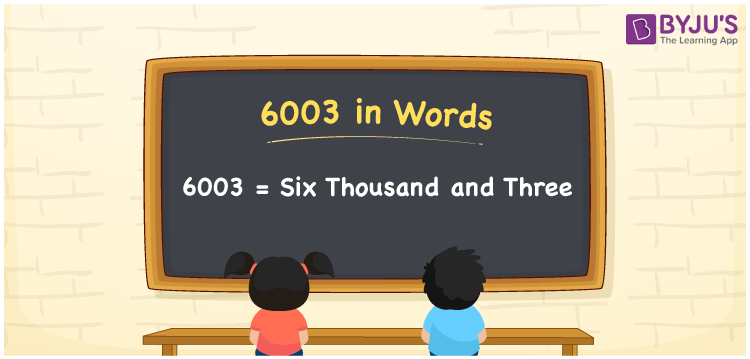# 6003 in words

6003 in words is written as Six Thousand and Three. 6003 represents the count or value. The article on Counting Numbers can give you an idea about count or counting. The number 6003 is used in expressions that relate to money, distance, length, year and others. Let us consider an example for 6003. ”A dog shelter aims to vaccinate Six Thousand and Three street dogs by the next quarter.”

 6003 in words Six Thousand and Three Six Thousand and Three in Numbers 6003

## 6003 in English Words## How to Write 6003 in Words?

We can convert 6003 to words using a place value chart. The number 6003 has 4 digits, so let’s make a chart that shows the place value up to 4 digits.

 Thousands Hundreds Tens Ones 6 0 0 3

Thus, we can write the expanded form as:

6 × Thousand + 0 × Hundred + 0 × Ten + 3 × One

= 6 × 1000 + 0 × 100 + 0 × 10 + 3 × 1

= 6003

= Six Thousand and Three.

6003 is the natural number that is succeeded by 6002 and preceded by 6004.

6003 in words – Six Thousand and Three.

Is 6003 an odd number? – Yes.

Is 6003 an even number? – No.

Is 6003 a perfect square number? – No.

Is 6003 a perfect cube number? – No.

Is 6003 a prime number? – No.

Is 6003 a composite number? – Yes.

## Solved Example

1. Write the number 6003 in expanded form

Solution: 6 × 1000 + 0 × 100 + 0 × 10 + 3 × 1

We can write 6003 = 6000 + 000 + 00 + 3

= 6 × 1000 + 0 × 100 + 0 × 10 + 3 × 1

## Frequently Asked Questions on 6003 in words

Q1

### How to write 6003 in words?

6003 in words is written as Six Thousand and Three.
Q2

### Is 6003 a perfect square number?

No. 6003 is not a perfect square number.
Q3

### Is 6003 a prime number?

No. 6003 is not a prime number.